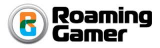# Points Library (ssk.points.*)

This is a general Lua library for creating lists of points `{ x = value, y = value }`.

These lists are used by the Line Factories, but may be useful in other contexts too.

# Creating a Points List

You can create a new points list with this function, which returns a points instance.

``````ssk.points.new( ... )
``````
• `...` - Optional sequence of x, y values to initialized the points list with.
``````
-- Create a points list with these points:
--  { x = 10, y = 10 }
--  { x = 20, y = 20 }
--  { x = 15, y = 35 }
--
local pointsList = ssk.points.new( 10, 10, 20, 20, 15, 35 )

``````

Tip: Points lists are numerically indexed tables, so you can do things like this:

``````
for 1 = 1, #pointsList do
print( "X @ index " .. i .. " == " .. tostring( pointsList[i].x ) )
print( "Y @ index " .. i .. " == " .. tostring( pointsList[i].y ) )
end

``````

Warning: Points lists cannot be saved to JSON because they have functions attached to them.

# Points Instance Methods

Points list instances have all of these methods:

| | |-------------:|:-------------| | `add(...)` | Add new points using x, y pairs (`...`) | | `insert(index, ...)` | Insert x, y pairs in list `...` to curent point list starting at `index`. | | `get(index)` | Returns point (as table) at `index` in list, or `nil` if no such `index` exists. | | `remove(index)` | Remove point (x, y pair) at `index` from list.| | `push(...)` | Add a new point(s) at end of list. | | `peek()` | Get point from end of list without removing it from the list. Returns `nil` if list is empty. | | `pop()` | Like `peek()`, but removes point from list at same time. | | `push_head(...)` | Add new point(s) to beginning of list. Synonym for `add( 1, ... )`. | | `peek_head()` | Get point from beginning (index 1) of the list. Returns `nil` if list is empty. | | `pop_head( )` | Like `peek_head()`, but removes point from list at same time. |

# Complete Points Example

``````
-- 1. Create blank list
local pointsList = ssk.points.new( )

-- 2.  Add points
pointsList:add( 15, 35 )

-- Points list is now:
--  { x = 15, y = 35 }

-- 3. Insert points at index 1
pointsList:insert( 1, 10, 10, 20, 20 )

-- Points list is now:
--  { x = 10, y = 10 }
--  { x = 20, y = 20 }
--  { x = 15, y = 35 }

-- 4. Pop Head

-- Points list is now:
--  { x = 20, y = 20 }
--  { x = 15, y = 35 }

-- 5. Pop Tail
pointsList:pop()

-- Points list is now:
--  { x = 20, y = 20 }

-- 6. Push Point to Tail
pointsList:push( 10, 10 )

-- Points list is now:
--  { x = 20, y = 20 }
--  { x = 10, y = 10 }

-- 7. Push Point to Head
pointsList:push_head( 15, 25 )

-- Points list is now:
--  { x = 15, y = 25 }
--  { x = 20, y = 20 }
--  { x = 10, y = 10 }

-- 8. Dump the Points
for i = 1, #pointsList do
table.dump(pointsList:get(i), nil, "Index: " .. tostring(i) )
end

``````

When executed, the above code prints (something like) this in the console:

``````
22:01:20.583  Table Dump:
22:01:20.583  -----
22:01:20.583  x (string)                     == 15 (number)
22:01:20.583  y (string)                     == 25 (number)
22:01:20.583  Index: 1-----
22:01:20.583
22:01:20.583  Table Dump:
22:01:20.583  -----
22:01:20.583  x (string)                     == 20 (number)
22:01:20.583  y (string)                     == 20 (number)
22:01:20.583  Index: 2-----
22:01:20.583
22:01:20.583  Table Dump:
22:01:20.583  -----
22:01:20.583  x (string)                     == 10 (number)
22:01:20.583  y (string)                     == 10 (number)
22:01:20.583  Index: 3-----

``````Copyright © Roaming Gamer, LLC. 2008-2016; All Rights Reserved